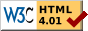## Dokumentation zu: m_values(E)``` SYNOPSIS mixed * m_values(mapping map) mixed * m_values(mapping map, int index) DESCRIPTION Returns an array with the values of mapping 'map'. If is given as a number between 0 and the width of the mapping, the values from the given column are returned, else the values of the first column. EXAMPLES mapping m = ([ "foo":1;2;3, "bar":4;5;6, "baz":7;8;9 ]) m_values(m) --> returns ({ 1, 4, 7 }) or some permutation thereof m_values(m, 0) --> returns ({ 1, 4, 7 }) (ditto) m_values(m, 1) --> returns ({ 2, 8, 9 }) (ditto) Note that exact order of the values in the resulting arrays is not specified, and may vary after any change to the mapping. The only guarantee given is that if m_indices() and m_values() are taken at the same time, the order of both results is identical. SEE ALSO mappingp(E), mkmapping(E), m_indices(E), m_add(E), m_delete(E), sizeof(E), widthof(E), unmkmapping(E) ``` Die Seite ist auch in Deutsch vorhanden.

Start » Magierhandbuch » Docu » Efun » M_values Letzte Generierung: 25.04.2021, 01:58mud@wl.mud.de